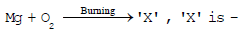Courses

# Atoms And Molecules - Class 9 Science (Compulsory Test)

## 30 Questions MCQ Test | Atoms And Molecules - Class 9 Science (Compulsory Test)

Description
This mock test of Atoms And Molecules - Class 9 Science (Compulsory Test) for Class 9 helps you for every Class 9 entrance exam. This contains 30 Multiple Choice Questions for Class 9 Atoms And Molecules - Class 9 Science (Compulsory Test) (mcq) to study with solutions a complete question bank. The solved questions answers in this Atoms And Molecules - Class 9 Science (Compulsory Test) quiz give you a good mix of easy questions and tough questions. Class 9 students definitely take this Atoms And Molecules - Class 9 Science (Compulsory Test) exercise for a better result in the exam. You can find other Atoms And Molecules - Class 9 Science (Compulsory Test) extra questions, long questions & short questions for Class 9 on EduRev as well by searching above.
QUESTION: 1

Solution:
QUESTION: 2

Solution:
QUESTION: 3

### The overall charge on an ionic compound is equal to -

Solution:
QUESTION: 4

The chemical formula of the copper nitrate

Solution:
QUESTION: 5

The number of carbon atoms in 1g of CaCO3 is-

Solution:
QUESTION: 6

The mass of a single atom of carbon is -

Solution:
QUESTION: 7

How many molecules are present in 9g of water -

Solution:
QUESTION: 8Solution:
QUESTION: 9

The formula of sulphuric acid is-

Solution:
QUESTION: 10

What is true about potassium chlorate -

Solution:
QUESTION: 11

The law of multiple proportions was proposed by :-

Solution:
QUESTION: 12

The weight of two elements which combine with each other are in the ratio of their:-

Solution:
QUESTION: 13

180 grams of water conatins ________ moles :-

Solution:
QUESTION: 14

What is the weight of 3 gram atoms of sulphur ?

Solution:
QUESTION: 15

How many gram atoms are present in 144 g of magnesium ?

Solution:
QUESTION: 16

How many moles of oxygen atoms are present in one mole of acetic acid ?

Solution:
QUESTION: 17

What is the number of particles in one mole of a substance ?

Solution:
QUESTION: 18

How many atoms and how many gram atoms are there in 10 grams of calcium ?

Solution:
QUESTION: 19

Calculate the weight of 0.1 mole of sodium carbonate :-

Solution:
QUESTION: 20

How many number of moles are present in 540 g of glucose?

Solution:
QUESTION: 21

Mass of one Avogadro's number of O atom is equal to -

Solution:
QUESTION: 22

Hydrogen reacts with oxygen to form water (H2O). The ratio between masses of Hydrogen and oxygen is

Solution:
QUESTION: 23

The mass of 1 u is -

Solution:
QUESTION: 24

Which of the following has highest intermolecular forces of attraction -

Solution:
QUESTION: 25

Which of the following is not correct regarding gases-

Solution:
QUESTION: 26

The Boiling point of water at normal atmospheric pressure is -

Solution:
QUESTION: 27

Avogadro's number represents the number, of atoms in-

Solution:
QUESTION: 28

Molecular mass of ozone is

Solution:
QUESTION: 29

Which of the following is not correct according to Dalton's atomic theory?

Solution:
QUESTION: 30

How much of lime can be obtained by burining 400 g of lime stone ?

Solution: